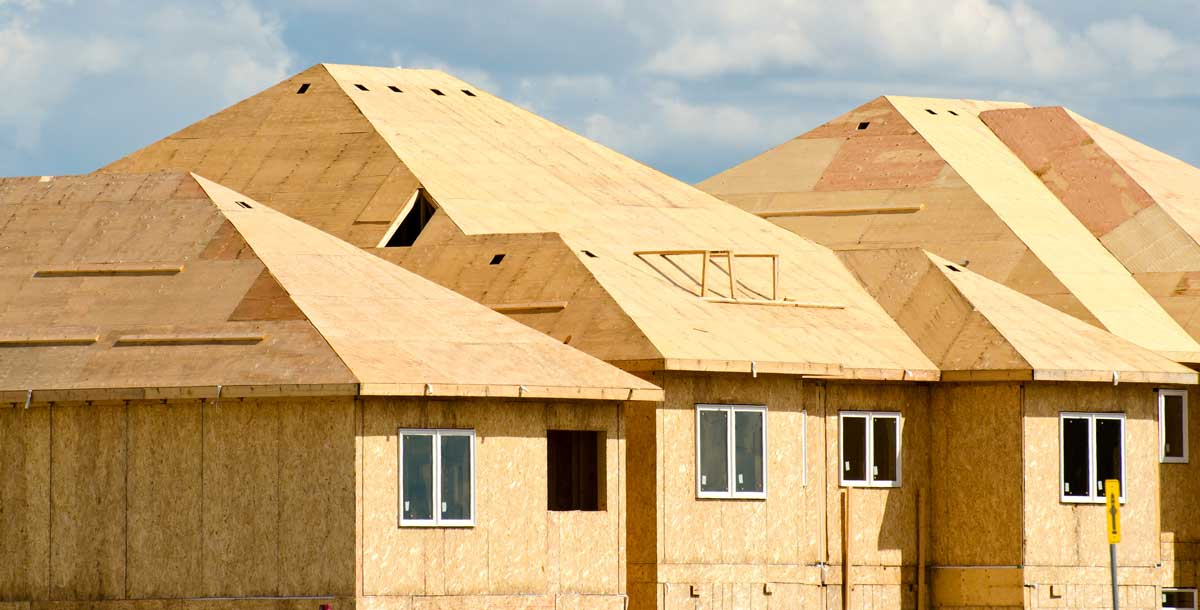# Roof Sheathing Calculator – Estimate the Plywood Needed for a Roof

Estimate how many sheets of 4×8 plywood are needed to sheath a roof, accounting for roof pitch.

## Plywood Estimate:

sheets
Learn how we calculated this below

## Find How Many Sheets of Plywood You Need to Sheath a RoofTo find the amount of plywood needed to cover a roof start by finding the area of the roof. Once the area is known, divide by the area of a 4×8 sheet to find how much sheathing is needed.

### Find the Area of a Roof

Multiply the length of the roof in feet by the width of the roof in feet to find the area of the roof in square feet. If you have multiple sections of roof find the area of each and add them together to find the total area.

If your roof is not flat and you cannot climb to the peak to get accurate width measurements then you can add a multiplier for the pitch of your roof.

Use our roof pitch calculator to find the pitch of your roof or find the pitch multiplier, then multiply the area of the footprint of your roof by the multiplier to find the actual area.

Alternatively, find the area of your roof using our roofing calculator, which can also estimate shingles and material needed for your project.

Get Free Project Estimates

Find Qualified Roofing Professionals in Your Area

For example, if your roof is 30 ft by 50 ft, then the area is 1,500 ft2.
30 × 50 = 1,500 ft2

If your roof has triangles and other shapes you’ll need to use a calculator to find the square footage.

### Calculate Sheathing Needed

Once you have the area you can divide by the area of a sheet of plywood to find the number of sheets needed. A 4×8 sheet of plywood is 32 ft2. So, dividing the area of the roof by 32 will give you the number of sheets needed.

For example, if your roof is 1,500 ft2 then it will take 47 sheets of plywood to sheath it.
1,500 ÷ 32 = 46.875 sheets

It will be a good idea to get an extra few sheets of plywood when ordering to allow for waste and off-cuts. Small scraps are often unusable to span joists and effectively cover the roof so extra material will save extra trips to the hardware store.